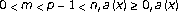# Entire Bounded Solutions for a Class of Quasilinear Elliptic Equations

## Abstract

We consider the problemwhereis not identically zero. Under the condition thatsatisfies (H), we show that there existssuch that the above-mentioned equation admits at least one solution for all. This extends the results of Laplace equation to the case of-Laplace equation.

## References

1. Herrero MA, Vázquez JL: On the propagation properties of a nonlinear degenerate parabolic equation. Communications in Partial Differential Equations 1982,7(12):1381–1402. 10.1080/03605308208820255

2. Esteban JR, Vázquez JL: On the equation of turbulent filtration in one-dimensional porous media. Nonlinear Analysis 1986,10(11):1303–1325. 10.1016/0362-546X(86)90068-4

3. Yang Z: Existence of positive bounded entire solutions for quasilinear elliptic equations. Applied Mathematics and Computation 2004,156(3):743–754. 10.1016/j.amc.2003.06.024

4. Guedda M, Véron L: Local and global properties of solutions of quasilinear elliptic equations. Journal of Differential Equations 1988,76(1):159–189. 10.1016/0022-0396(88)90068-X

5. Guo ZM: Existence and uniqueness of positive radial solutions for a class of quasilinear elliptic equations. Applicable Analysis 1992,47(2–3):173–189.

6. Guo ZM: Some existence and multiplicity results for a class of quasilinear elliptic eigenvalue problems. Nonlinear Analysis 1992,18(10):957–971. 10.1016/0362-546X(92)90132-X

7. Guo ZM, Webb JRL: Uniqueness of positive solutions for quasilinear elliptic equations when a parameter is large. Proceedings of the Royal Society of Edinburgh. Section A. Mathematics 1994,124(1):189–198. 10.1017/S0308210500029280

8. Lu Q, Yang Z, Twizell EH: Existence of entire explosive positive solutions of quasi-linear elliptic equations. Applied Mathematics and Computation 2004,148(2):359–372. 10.1016/S0096-3003(02)00852-4

9. Bognár G, Drábek P: The-Laplacian equation with superlinear and supercritical growth, multiplicity of radial solutions. Nonlinear Analysis 2005,60(4):719–728. 10.1016/j.na.2004.09.047

10. Prashanth S, Sreenadh K: Multiplicity of positive solutions for-Laplace equation with superlinear-type nonlinearity. Nonlinear Analysis 2004,56(6):867–878. 10.1016/j.na.2003.10.026

11. Brezis H, Kamin S: Sublinear elliptic equations in. Manuscripta Mathematica 1992,74(1):87–106. 10.1007/BF02567660

12. Ambrosetti A, Brezis H, Cerami G: Combined effects of concave and convex nonlinearities in some elliptic problems. Journal of Functional Analysis 1994,122(2):519–543. 10.1006/jfan.1994.1078

13. Brezis H, Oswald L: Remarks on sublinear elliptic equations. Nonlinear Analysis 1986,10(1):55–64. 10.1016/0362-546X(86)90011-8

14. Bartsch T, Willem M: On an elliptic equation with concave and convex nonlinearities. Proceedings of the American Mathematical Society 1995,123(11):3555–3561. 10.1090/S0002-9939-1995-1301008-2

15. Ye D, Zhou F: Invariant criteria for existence of bounded positive solutions. Discrete and Continuous Dynamical Systems. Series A 2005,12(3):413–424.

16. El Mabrouk K: Entire bounded solutions for a class of sublinear elliptic equations. Nonlinear Analysis 2004,58(1–2):205–218. 10.1016/j.na.2004.01.004

## Author information

Authors

### Corresponding author

Correspondence to Zuodong Yang.

## Rights and permissions

Reprints and Permissions

Yang, Z., Xu, B. Entire Bounded Solutions for a Class of Quasilinear Elliptic Equations. Bound Value Probl 2007, 016407 (2007). https://doi.org/10.1155/2007/16407

• Accepted:

• Published:

• DOI: https://doi.org/10.1155/2007/16407

### Keywords

• Differential Equation
• Partial Differential Equation
• Ordinary Differential Equation
• Functional Equation
• Elliptic Equation